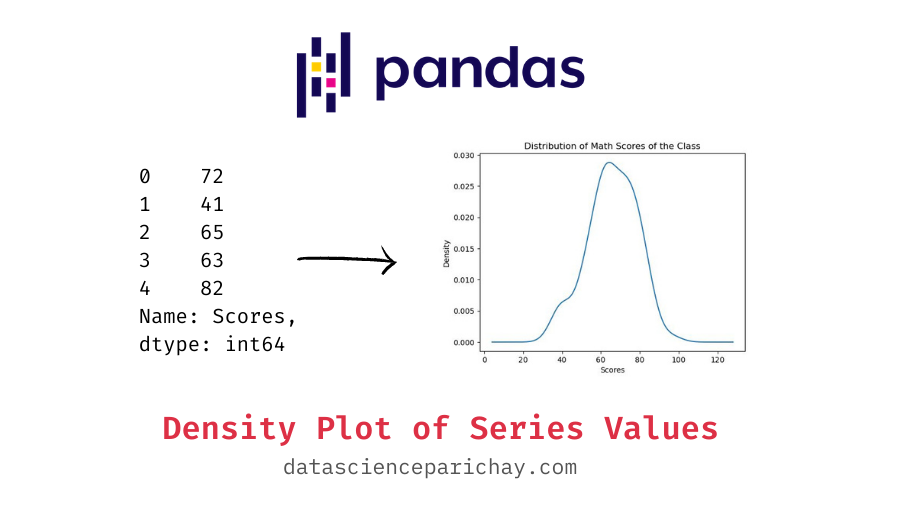# Create a Density Plot from Pandas Series Values

In this tutorial, we will look at how to create a density plot of a pandas series values.

To plot a pandas series, you can use the pandas series `plot()` function. It plots a line chart of the series values by default but you can specify the type of chart to plot using the `kind` parameter. To plot a density chart, pass `'density'` to the `kind` parameter. The following is the syntax:

```# density plot using pandas series plot()
s.plot(kind='density')```

Here, s is the pandas series you want to plot. The pandas series `plot()` function returns a matplotlib axes object to which you can add additional formatting.

Let’s look at some examples of plotting a pandas series values as a density chart. First, we’ll create a sample pandas series which we will be using throughout this tutorial.

```import pandas as pd

# scores in the Math class
math_scores = pd.Series(data=[72, 41, 65, 63, 82, 63, 51, 57, 39, 63,
62, 68, 52, 76, 62, 73, 72, 73, 71, 62,
76, 53, 71, 79, 77, 35, 65, 59, 58, 70,
73, 69, 59, 75, 73, 63, 65, 81, 46, 59,
53, 71, 79, 80, 60, 60, 64, 40, 73, 75,
68, 58, 81, 65, 55, 62, 82, 47, 85, 62,
39, 77, 82, 78, 57, 58, 72, 75, 65, 68,
86, 49, 39, 64, 54, 68, 85, 77, 62, 53,
52, 76, 80, 84, 69, 61, 69, 65, 89, 97,
71, 61, 77, 40, 83, 52, 78, 54, 64, 58],
name='Scores')

Output:

```0    72
1    41
2    65
3    63
4    82
Name: Scores, dtype: int64```

You can see the top five values of the series object above. We now have a pandas series containing the scores of students in a Math class.

To create a density plot, we’ll pass `kind='density'` to the pandas series `plot()` function. For example, let’s see its usage on the “math_scores” series created above.

`math_scores.plot(kind='density')`

The above plot shows the distribution of values in the series which closely resembles a bell curve. Notice, a large number of students got scores closer to the mean.

📚 Data Science Programs By Skill Level

Introductory

Intermediate ⭐⭐⭐

🔎 Find Data Science Programs 👨‍💻 111,889 already enrolled

Disclaimer: Data Science Parichay is reader supported. When you purchase a course through a link on this site, we may earn a small commission at no additional cost to you. Earned commissions help support this website and its team of writers.

For more on the pandas series plot() function, refer to its documentation.

You can also customize the formatting of the chart. For instance, you can add the axes labels, chart title, change colors and fonts, etc. Since the returned plot is a matplotlib axes object, you can apply any formatting that would work with matplotlib charts.

Let’s go ahead and add the x-axis label and title to our plot.

```# create the density plot
ax = math_scores.plot(kind='density')
# set the x-axis label
ax.set_xlabel("Scores")
# set the title
ax.set_title("Distribution of Math Scores of the Class")```

Output:

You can see the above chart has “Scores” as its x-axis label, and “Distribution of Math Scores of the Class” as its title.

With this, we come to the end of this tutorial. The code examples and results presented in this tutorial have been implemented in a Jupyter Notebook with a python (version 3.8.3) kernel having pandas version 1.0.5

•